PROC LOGISTIC Statement

PROC LOGISTIC <options> ;

The PROC LOGISTIC statement invokes the LOGISTIC procedure. Optionally, it identifies input and output data sets, suppresses the display of results, and controls the ordering of the response levels. Table 54.1 summarizes the options available in the PROC LOGISTIC statement.

Table 54.1: PROC LOGISTIC Statement Options

Option

Description

Input/Output Data Set Options

Displays the estimated covariance matrix in the OUTEST= data set

Names the input SAS data set

Specifies the initial estimates SAS data set

Specifies the model information SAS data set

Does not save covariance matrix in the OUTMODEL= data set

Specifies the design matrix output SAS data set

Outputs the design matrix only

Specifies the parameter estimates output SAS data set

Specifies the model output data set for scoring

Response and CLASS Variable Options

Reverses the sort order of the response variable

Specifies the maximum length of effect names

Specifies the sort order of the response variable

Truncates class level names

Displayed Output Options

Specifies the significance level for confidence intervals

Suppresses all displayed output

Specifies options for plots

Displays descriptive statistics

Large Data Set Option

Does not copy the input SAS data set for internal computations

Control of Other Statement Options

Performs exact analysis only

Specifies global options for EXACT statements

Specifies global options for ROC statements

ALPHA=number

specifies the level of significancefor% confidence intervals. The value number must be between 0 and 1; the default value is 0.05, which results in 95% intervals. This value is used as the default confidence level for limits computed by the following options:

Statement

Options

CONTRAST

EXACT

MODEL

ODDSRATIO

OUTPUT

PROC LOGISTIC

PLOTS=EFFECT(CLBAR CLBAND)

ROCCONTRAST

SCORE

You can override the default in most of these cases by specifying the ALPHA= option in the separate statements.

COVOUT

adds the estimated covariance matrix to the OUTEST= data set. For the COVOUT option to have an effect, the OUTEST= option must be specified. See the section OUTEST= Output Data Set for more information.

DATA=SAS-data-set

names the SAS data set containing the data to be analyzed. If you omit the DATA= option, the procedure uses the most recently created SAS data set. The INMODEL= option cannot be specified with this option.

DESCENDING
DESC

reverses the sort order for the levels of the response variable. If both the DESCENDING and ORDER= options are specified, PROC LOGISTIC orders the levels according to the ORDER= option and then reverses that order. This option has the same effect as the response variable option DESCENDING in the MODEL statement. See the section Response Level Ordering for more detail.

EXACTONLY

requests only the exact analyses. The asymptotic analysis that PROC LOGISTIC usually performs is suppressed.

EXACTOPTIONS (options)

specifies options that apply to every EXACT statement in the program. The available options are summarized here, and full descriptions are available in the EXACTOPTIONS statement.

Option

Description

Adds the observed sufficient statistic to the sampled exact distribution

Builds every distribution for sampling

Specifies the comparison fuzz for partial sums of sufficient statistics

Specifies the maximum time allowed in seconds

Specifies the DIRECT, NETWORK, or NETWORKMC algorithm

Specifies the number of Monte Carlo samples

Uses disk space

Specifies the initial seed for sampling

Specifies the sampling interval for printing a status line

Specifies the time interval for printing a status line

INEST=SAS-data-set

names the SAS data set that contains initial estimates for all the parameters in the model. If BY-group processing is used, it must be accommodated in setting up the INEST= data set. See the section INEST= Input Data Set for more information.

INMODEL=SAS-data-set

specifies the name of the SAS data set that contains the model information needed for scoring new data. This INMODEL= data set is the OUTMODEL= data set saved in a previous PROC LOGISTIC call. The OUTMODEL= data set should not be modified before its use as an INMODEL= data set.

The DATA= option cannot be specified with this option; instead, specify the data sets to be scored in the SCORE statements. FORMAT statements are not allowed when the INMODEL= data set is specified; variables in the DATA= and PRIOR= data sets in the SCORE statement should be formatted within the data sets.

You can specify the BY statement provided that the INMODEL= data set is created under the same BY-group processing.

The CLASS, EFFECT, EFFECTPLOT, ESTIMATE, EXACT, LSMEANS, LSMESTIMATE, MODEL, OUTPUT, ROC, ROCCONTRAST, SLICE, STORE, TEST, and UNIT statements are not available with the INMODEL= option.

MULTIPASS

forces the procedure to reread the DATA= data set as needed rather than require its storage in memory or in a temporary file on disk. By default, the data set is cleaned up and stored in memory or in a temporary file. This option can be useful for large data sets. All exact analyses are ignored in the presence of the MULTIPASS option. If a STRATA statement is specified, then the data set must first be grouped or sorted by the strata variables.

NAMELEN=n

specifies the maximum length of effect names in tables and output data sets to be n characters, where n is a value between 20 and 200. The default length is 20 characters.

NOCOV

specifies that the covariance matrix not be saved in the OUTMODEL= data set. The covariance matrix is needed for computing the confidence intervals for the posterior probabilities in the OUT= data set in the SCORE statement. Specifying this option will reduce the size of the OUTMODEL= data set.

NOPRINT

suppresses all displayed output. Note that this option temporarily disables the Output Delivery System (ODS); see Chapter 20: Using the Output Delivery System, for more information.

ORDER=DATA | FORMATTED | FREQ | INTERNAL
RORDER=DATA | FORMATTED | INTERNAL

specifies the sort order for the levels of the response variable. See the response variable option ORDER= in the MODEL statement for more information. For ordering of CLASS variable levels, see the ORDER= option in the CLASS statement.

OUTDESIGN=SAS-data-set

specifies the name of the data set that contains the design matrix for the model. The data set contains the same number of observations as the corresponding DATA= data set and includes the response variable (with the same format as in the DATA= data set), the FREQ variable, the WEIGHT variable, the OFFSET= variable, and the design variables for the covariates, including the Intercept variable of constant value 1 unless the NOINT option in the MODEL statement is specified.

OUTDESIGNONLY

suppresses the model fitting and creates only the OUTDESIGN= data set. This option is ignored if the OUTDESIGN= option is not specified.

OUTEST=SAS-data-set

creates an output SAS data set that contains the final parameter estimates and, optionally, their estimated covariances (see the preceding COVOUT option). The output data set also includes a variable named _LNLIKE_, which contains the log likelihood. See the section OUTEST= Output Data Set for more information.

OUTMODEL=SAS-data-set

specifies the name of the SAS data set that contains the information about the fitted model. This data set contains sufficient information to score new data without having to refit the model. It is solely used as the input to the INMODEL= option in a subsequent PROC LOGISTIC call. The OUTMODEL= option is not available with the STRATA statement. Information in this data set is stored in a very compact form, so you should not modify it manually.

Note: The STORE statement can also be used to save your model. See the section STORE Statement for more information.

PLOTS <(global-plot-options)><=plot-request<(options)>>
PLOTS <(global-plot-options)> =(plot-request<(options)>< …plot-request<(options)>>)

controls the plots produced through ODS Graphics. When you specify only one plot-request, you can omit the parentheses from around the plot-request. For example:

PLOTS = ALL
PLOTS = (ROC EFFECT INFLUENCE(UNPACK))
PLOTS(ONLY) = EFFECT(CLBAR SHOWOBS)

ODS Graphics must be enabled before plots can be requested. For example:

ods graphics on;
proc logistic plots=all;
model y=x;
run;
ods graphics off;

For more information about enabling and disabling ODS Graphics, see the section Enabling and Disabling ODS Graphics in Chapter 21: Statistical Graphics Using ODS.

If the PLOTS option is not specified or is specified with no plot-requests, then graphics are produced by default in the following situations:

• If the INFLUENCE or IPLOTS option is specified in the MODEL statement, then the line-printer plots are suppressed, and the INFLUENCE plots are produced unless the MAXPOINTS= cutoff is exceeded.

• If you specify the OUTROC= option in the MODEL statement, then ROC curves are produced. If you also specify a SELECTION= method, then an overlaid plot of all the ROC curves for each step of the selection process is displayed.

• If the OUTROC= option is specified in a SCORE statement, then the ROC curve for the scored data set is displayed.

• If you specify ROC statements, then an overlaid plot of the ROC curves for the model (or the selected model if a SELECTION= method is specified) and for all the ROC statement models is displayed.

• If an odds ratio table is produced, then a plot of the odds ratios and their confidence limits is displayed. These plots correspond to the default odds ratio table and the tables produced by the CLODDS= option in the MODEL statement and the tables produced by the ODDSRATIO statement.

For general information about ODS Graphics, see Chapter 21: Statistical Graphics Using ODS.

The following global-plot-options are available:

LABEL

displays a label on diagnostic plots to aid in identifying the outlying observations. This option enhances the plots produced by the DFBETAS, DPC, INFLUENCE, LEVERAGE, and PHAT options. If an ID statement is specified, then the plots are labeled with the ID variables. Otherwise, the observation number is displayed.

MAXPOINTS=NONE | number

suppresses the plots produced by the DFBETAS, DPC, INFLUENCE, LEVERAGE, and PHAT options if there are more than number observations. Also, observations are not displayed on the EFFECT plots when the cutoff is exceeded. The default is MAXPOINTS=5000. The cutoff is ignored if you specify MAXPOINTS=NONE.

ONLY

specifically requested plot-requests are displayed.

UNPACKPANELS | UNPACK

suppresses paneling. By default, multiple plots can appear in some output panels. Specify UNPACKPANEL to display each plot separately.

The following plot-requests are available:

ALL

produces all appropriate plots. You can specify other options with ALL. For example, to display all plots and unpack the DFBETAS plots you can specify plots=(all dfbetas(unpack)).

DFBETAS <(UNPACK)>

displays plots of DFBETAS versus the case (observation) number. This displays the statistics generated by the DFBETAS=_ALL_ option in the OUTPUT statement. The UNPACK option displays the plots separately. See Output 54.6.5 for an example of this plot.

DPC<(UNPACK)>

displays plots of DIFCHISQ and DIFDEV versus the predicted event probability, and colors the markers according to the value of the confidence interval displacement C. The UNPACK option displays the plots separately. See Output 54.6.8 for an example of this plot.

EFFECT<(effect-options)>

displays and enhances the effect plots for the model. For more information about effect plots and the available effect-options, see the section PLOTS=EFFECT Plots.

Note: The EFFECTPLOT statement provides you with much of the same functionality and more options for creating effect plots. See Outputs Output 54.2.11, Output 54.3.5, Output 54.4.8, Output 54.7.4, and Output 54.15.4 for examples of effect plots.

INFLUENCE<(UNPACK | STDRES)>

displays index plots of RESCHI, RESDEV, leverage, confidence interval displacements C and CBar, DIFCHISQ, and DIFDEV. These plots are produced by default when any plot-request is specified and the MAXPOINTS= cutoff is not exceeded. The UNPACK option displays the plots separately. The STDRES option also displays index plots of STDRESCHI, STDRESDEV, and RESLIK. See Outputs Output 54.6.3 and Output 54.6.4 for examples of these plots.

LEVERAGE<(UNPACK)>

displays plots of DIFCHISQ, DIFDEV, confidence interval displacement C, and the predicted probability versus the leverage. The UNPACK option displays the plots separately. See Output 54.6.7 for an example of this plot.

NONE

suppresses all plots.

ODDSRATIO <(oddsratio-options)>

displays and enhances the odds ratio plots for the model. For more information about odds ratio plots and the available oddsratio-options, see the section Odds Ratio Plots. See Outputs Figure 54.7,Output 54.2.9, Output 54.3.3, and Output 54.4.5 for examples of this plot.

PHAT<(UNPACK)>

displays plots of DIFCHISQ, DIFDEV, confidence interval displacement C, and leverage versus the predicted event probability. The UNPACK option displays the plots separately. See Output 54.6.6 for an example of this plot.

ROC<(ID<=keyword>)>

displays the ROC curve. If you also specify a SELECTION= method, then an overlaid plot of all the ROC curves for each step of the selection process is displayed. If you specify ROC statements, then an overlaid plot of the model (or the selected model if a SELECTION= method is specified) and the ROC statement models is displayed. If the OUTROC= option is specified in a SCORE statement, then the ROC curve for the scored data set is displayed.

The ID= option labels certain points on the ROC curve. Typically, the labeled points are closest to the upper-left corner of the plot, and points directly below or to the right of a labeled point are suppressed. This option is identical to, and has the same keywords as, the ID= suboption of the ROCOPTIONS option.

See Output 54.7.3 and Example 54.8 for examples of these ROC plots.

ROCOPTIONS (options)

specifies options that apply to every model specified in a ROC statement. Some of these options also apply to the SCORE statement. The following options are available:

ALPHA=number

sets the significance level for creating confidence limits of the areas and the pairwise differences. The ALPHA= value specified in the PROC LOGISTIC statement is the default. If neither ALPHA= value is specified, then ALPHA=0.05 by default.

EPS=value

is an alias for the ROCEPS= option in the MODEL statement. This value is used to determine which predicted probabilities are equal. The default value is the square root of the machine epsilon, which is about 1E–8.

ID<=keyword>

displays labels on certain points on the individual ROC curves and also on the SCORE statement’s ROC curve. This option overrides the ID= suboption of the PLOTS=ROC option. If several observations lie at the same place on the ROC curve, the value for the last observation is displayed. If you specify the ID option with no keyword, any variables that are listed in the ID statement are used. If no ID statement is specified, the observation number is displayed. The following keywords are available:

PROB

displays the model predicted probability.

OBS

displays the (last) observation number.

SENSIT

displays the true positive fraction (sensitivity).

1MSPEC

displays the false positive fraction (1–specificity).

FALPOS

displays the fraction of nonevents that are predicted as events.

FALNEG

displays the fraction of events that are predicted as nonevents.

POSPRED

displays the positive predictive value (1–FALPOS).

NEGPRED

displays the negative predictive value (1–FALNEG).

MISCLASS

displays the misclassification rate.

ID

displays the ID variables.

The SENSIT, 1MSPEC, FALPOS, and FALNEG statistics are defined in the section Receiver Operating Characteristic Curves. The misclassification rate is the number of events that are predicted as nonevents and the number of nonevents that are predicted as events as calculated by using the given cutpoint (predicted probability) divided by the number of observations. If the PEVENT= option is also specified, then FALPOS and FALNEG are computed using the first PEVENT= value and Bayes’ theorem, as discussed in the section Predicted Probability of an Event for Classification.

NODETAILS

suppresses the display of the model fitting information for the models specified in the ROC statements.

OUT=SAS-data-set-name

is an alias for the OUTROC= option in the MODEL statement.

WEIGHTED

uses frequencyweight in the ROC computations (Izrael et al., 2002) instead of just frequency. Typically, weights are considered in the fit of the model only, and hence are accounted for in the parameter estimates. The Association of Predicted Probabilities and Observed Responses table uses frequency (unless the BINWIDTH=0 option is also specified on the MODEL statement), and is suppressed when ROC comparisons are performed. This option also affects SCORE statement ROC and AUC computations.

SIMPLE

displays simple descriptive statistics (mean, standard deviation, minimum and maximum) for each continuous explanatory variable. For each CLASS variable involved in the modeling, the frequency counts of the classification levels are displayed. The SIMPLE option generates a breakdown of the simple descriptive statistics or frequency counts for the entire data set and also for individual response categories.

TRUNCATE

determines class levels by using no more than the first 16 characters of the formatted values of CLASS, response, and strata variables. When formatted values are longer than 16 characters, you can use this option to revert to the levels as determined in releases previous to SAS 9.0. This option invokes the same option in the CLASS statement.

PLOTS=EFFECT Plots

Only one PLOTS=EFFECT plot is produced by default; you must specify other effect-options to produce multiple plots. For binary response models, the following plots are produced when an EFFECT option is specified with no effect-options:

• If you only have continuous covariates in the model, then a plot of the predicted probability versus the first continuous covariate fixing all other continuous covariates at their means is displayed. See Output 54.7.4 for an example with one continuous covariate.

• If you only have classification covariates in the model, then a plot of the predicted probability versus the first CLASS covariate at each level of the second CLASS covariate, if any, holding all other CLASS covariates at their reference levels is displayed.

• If you have CLASS and continuous covariates, then a plot of the predicted probability versus the first continuous covariate at up to 10 cross-classifications of the CLASS covariate levels, while fixing all other continuous covariates at their means and all other CLASS covariates at their reference levels, is displayed. For example, if your model has four binary covariates, there are 16 cross-classifications of the CLASS covariate levels. The plot displays the 8 cross-classifications of the levels of the first three covariates while the fourth covariate is fixed at its reference level.

For polytomous response models, similar plots are produced by default, except that the response levels are used in place of the CLASS covariate levels. Plots for polytomous response models involving OFFSET= variables with multiple values are not available.

The following effect-options specify the type of graphic to produce:

AT(variable=value-list | ALL<...variable=value-list | ALL>)

specifies fixed values for a covariate. For continuous covariates, you can specify one or more numbers in the value-list. For classification covariates, you can specify one or more formatted levels of the covariate enclosed in single quotes (for example, A=’cat’ ’dog’), or you can specify the keyword ALL to select all levels of the classification variable. You can specify a variable at most once in the AT option. By default, continuous covariates are set to their means when they are not used on an axis, while classification covariates are set to their reference level when they are not used as an X=, SLICEBY=, or PLOTBY= effect. For example, for a model that includes a classification variable A={cat,dog} and a continuous covariate X, specifying AT(A=’cat’ X=7 9) will set A to 'cat' when A does not appear in the plot. When X does not define an axis it first produces plots setting X = 7 and then produces plots setting X = 9. Note in this example that specifying AT( A=ALL ) is the same as specifying the PLOTBY=A option.

FITOBSONLY

computes the predicted values only at the observed data. If the FITOBSONLY option is omitted and the X-axis variable is continuous, the predicted values are computed at a grid of points extending slightly beyond the range of the data (see the EXTEND= option for more information). If the FITOBSONLY option is omitted and the X-axis effect is categorical, the predicted values are computed at all possible categories.

INDIVIDUAL

displays the individual probabilities instead of the cumulative probabilities. This option is available only with cumulative models, and it is not available with the LINK option.

displays the linear predictors instead of the probabilities on the Y axis. For example, for a binary logistic regression, the Y axis will be displayed on the logit scale. The INDIVIDUAL and POLYBAR options are not available with the LINK option.

PLOTBY=effect

displays an effect plot at each unique level of the PLOTBY= effect. You can specify effect as one CLASS variable or as an interaction of classification covariates. For polytomous-response models, you can also specify the response variable as the lone PLOTBY= effect. For nonsingular parameterizations, the complete cross-classification of the CLASS variables specified in the effect define the different PLOTBY= levels. When the GLM parameterization is used, the PLOTBY= levels can depend on the model and the data.

SLICEBY=effect

displays predicted probabilities at each unique level of the SLICEBY= effect. You can specify effect as one CLASS variable or as an interaction of classification covariates. For polytomous-response models, you can also specify the response variable as the lone SLICEBY= effect. For nonsingular parameterizations, the complete cross-classification of the CLASS variables specified in the effect define the different SLICEBY= levels. When the GLM parameterization is used, the SLICEBY= levels can depend on the model and the data.

X=effect
X=(effect...effect)

specifies effects to be used on the X axis of the effect plots. You can specify several different X axes: continuous variables must be specified as main effects, while CLASS variables can be crossed. For nonsingular parameterizations, the complete cross-classification of the CLASS variables specified in the effect define the axes. When the GLM parameterization is used, the X= levels can depend on the model and the data. The response variable is not allowed as an effect.

Note: Any variable not specified in a SLICEBY= or PLOTBY= option is available to be displayed on the X axis. A variable can be specified in at most one of the SLICEBY=, PLOTBY=, and X= options.

The following effect-options enhance the graphical output:

ALPHA=number

specifies the size of the confidence limits. The ALPHA= value specified in the PROC LOGISTIC statement is the default. If neither ALPHA= value is specified, then ALPHA=0.05 by default.

CLBAND<=YES | NO>

displays confidence limits on the plots. This option is not available with the INDIVIDUAL option. If you have CLASS covariates on the X axis, then error bars are displayed (see the CLBAR option) unless you also specify the CONNECT option.

CLBAR

displays the error bars on the plots when you have CLASS covariates on the X axis; if the X axis is continuous, then this invokes the CLBAND option. For polytomous-response models with CLASS covariates only and with the POLYBAR option specified, the stacked bar charts are replaced by side-by-side bar charts with error bars.

CLUSTER<=percent>

displays the levels of the SLICEBY= effect in a side-by-side fashion instead of stacking them. This option is available when you have CLASS covariates on the X axis. You can specify percent as a percentage of half the distance between X levels. The percent value must be between 0.1 and 1; the default percent depends on the number of X levels and the number of SLICEBY= levels. Default clustering can be removed by specifying the NOCLUSTER option.

CONNECT<=YES | NO>
JOIN<=YES | NO>

connects the predicted values with a line. This option is available when you have CLASS covariates on the X axis. Default connecting lines can be suppressed by specifying the NOCONNECT option.

EXTEND=value

extends continuous X axes by a factor of value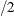in each direction. By default, EXTEND=0.2.

MAXATLEN=length

specifies the maximum number of characters used to display the levels of all the fixed variables. If the text is too long, it is truncated and ellipses () are appended. By default, length is equal to its maximum allowed value, 256.

NOCLUSTER

prevents clustering of the levels of the SLICEBY= effect. This option is available when you have CLASS covariates on the X axis.

NOCONNECT

removes the line that connects the predicted values. This option is available when you have CLASS covariates on the X axis.

POLYBAR

replaces scatter plots of polytomous response models with bar charts. This option has no effect on binary-response models, and it is overridden by the CONNECT option. By default, the X axis is chosen to be a crossing of available classification variables so that there are no more than 16 levels; if no such crossing is possible then the first available classification variable is used. You can override this default by specifying the X= option.

SHOWOBS<=YES | NO>

displays observations on the plot when the MAXPOINTS= cutoff is not exceeded. For events/trials notation, the observed proportions are displayed; for single-trial binary-response models, the observed events are displayed at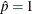and the observed nonevents are displayed at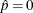. For polytomous response models the predicted probabilities at the observed values of the covariate are computed and displayed.

YRANGE=(<min><,max>)

displays the Y axis as [min,max]. Note that the axis might extend beyond your specified values. By default, the entire Y axis, [0,1], is displayed for the predicted probabilities. This option is useful if your predicted probabilities are all contained in some subset of this range.

Odds Ratio Plots

The odds ratios and confidence limits from the default Odds Ratio Estimates table and from the tables produced by the CLODDS= option or the ODDSRATIO statement can be displayed in a graphic. If you have many odds ratios, you can produce multiple graphics, or panels, by displaying subsets of the odds ratios. Odds ratios with duplicate labels are not displayed. See Outputs Output 54.2.9 and Output 54.3.3 for examples of odds ratio plots.

The following oddsratio-options modify the default odds ratio plot:

CLDISPLAY=SERIF | LINE | BAR<width>

controls the look of the confidence limit error bars. The default CLDISPLAY=SERIF displays the confidence limits as lines with serifs, CLDISPLAY=LINE removes the serifs from the error bars, and CLDISPLAY=BAR <width> displays the limits with a bar of width equal to the size of the marker. You can control the width of the bars and the size of the marker by specifying the width value as a percentage of the distance between the bars, 0
width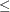1.

Note: Your bar might disappear with small values of width.

DOTPLOT

displays dotted gridlines on the plot.

GROUP

displays the odds ratios in panels defined by the ODDSRATIO statements. The NPANELPOS= option is ignored when this option is specified.

LOGBASE=2 | E | 10

displays the odds ratio axis on the specified log scale.

NPANELPOS=n

breaks the plot into multiple graphics having at most |n| odds ratios per graphic. If n is positive, then the number of odds ratios per graphic is balanced; but if n is negative, then no balancing of the number of odds ratios takes place. By default, n = 0 and all odds ratios are displayed in a single plot. For example, suppose you want to display 21 odds ratios. Then specifying NPANELPOS=20 displays two plots, the first with 11 odds ratios and the second with 10; but specifying NPANELPOS=-20 displays 20 odds ratios in the first plot and only 1 odds ratio in the second.

ORDER=ASCENDING | DESCENDING

displays the odds ratios in sorted order. By default the odds ratios are displayed in the order in which they appear in the corresponding table.

RANGE=(<min><,max>) | CLIP

specifies the range of the displayed odds ratio axis. The RANGE=CLIP option has the same effect as specifying the minimum odds ratio as min and the maximum odds ratio as max. By default, all odds ratio confidence intervals are displayed.

TYPE=HORIZONTAL | HORIZONTALSTAT | VERTICAL | VERTICALBLOCK

controls the look of the graphic. The default TYPE=HORIZONTAL option places the odds ratio values on the X axis, while the TYPE=HORIZONTALSTAT option also displays the values of the odds ratios and their confidence limits on the right side of the graphic. The TYPE=VERTICAL option places the odds ratio values on the Y axis, while the TYPE=VERTICALBLOCK option (available only with the CLODDS= option) places the odds ratio values on the Y axis and puts boxes around the labels.